﻿ Spherical Shell and Hemispherical Head Under External Pressure – Oil & Gas Industry Technology Updates

## Spherical Shell and Hemispherical Head Under External Pressure

The ASME Code procedure is as follows:
1. Calculate the value of A using the equation: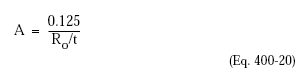where:
Ro is the outside radius of the sphere.
2. Find the value of B from the Code material/temperature chart in Appendix 5.
3. Calculate the maximum allowable external pressure: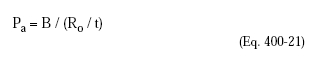or for values of A falling to the left of the applicable temperature line: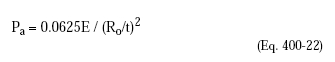Using the same example:
Ro = 48.5 in.
t = 0.125 in.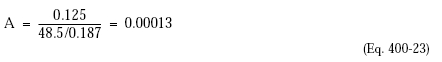From Figure UCS-28.2 B = 10,500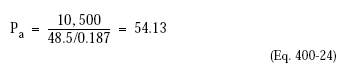The hemispherical head is good for the external design pressure of 54 psi.